Function Repository Resource:

# CosDegree

Compute the cosine of an angle given in degrees

Contributed by: Paco Jain (Wolfram Research)
 ResourceFunction["CosDegree"][x] computes the cosine of an angle x, measured in degrees.

## Details and Options

Mathematical function, suitable for both symbolic and numerical manipulation.
For certain special arguments, ResourceFunction["CosDegree"] automatically evaluates to exact values.
ResourceFunction["CosDegree"] can be evaluated to arbitrary numerical precision.

## Examples

### Basic Examples (2)

Compute the cosine of an angle:

 In:=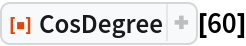Out=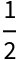Plot over a given range of angles:

 In:=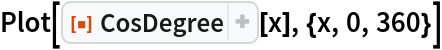Out=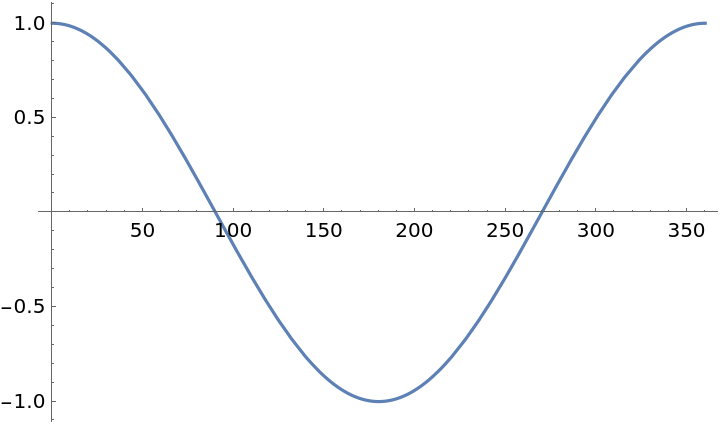### Scope (2)

Evaluate numerically:

 In:=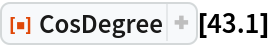Out=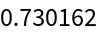Evaluate to high precision:

 In:=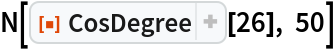Out=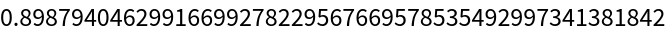### Applications (1)

Draw a circle:

 In:=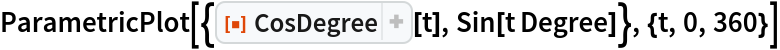Out=## Requirements

Wolfram Language 11.3 (March 2018) or above

## Version History

• 1.0.0 – 07 November 2018